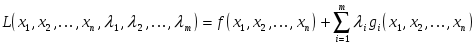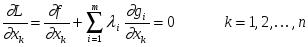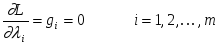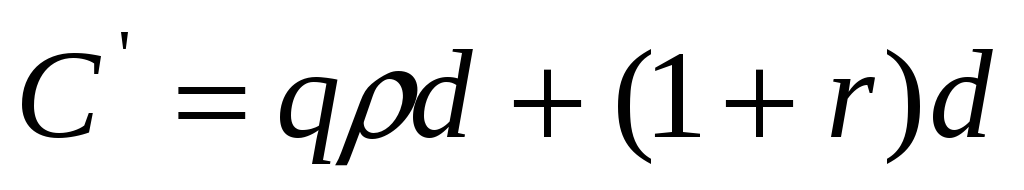# B. ceranic, Research Fellow and C. Fryer, Head of Department

 Page 1/7 Date 23.04.2018 Size 0.68 Mb.
Sensitivity Analysis and Optimum Design Curves for the Minimum Cost Design of Singly and Doubly Reinforced Concrete Beams

## B. CERANIC, Research Fellow and C. FRYER, Head of Department

School of Engineering, University of Derby, Kedleston Site, Kedleston Road,

Derby DE22 1GB, United Kingdom

## Abstract

This paper reports on the application of the Lagrangian Multiplier Method (LMM) to the minimum cost design of both singly and doubly reinforced concrete rectangular beams under limit state design conditions. Cost objective functions and stress constraints are derived and implemented within the optimisation method. Cost sensitivity analysis, detailed testing and comparisons with conventional design office methods are performed and the results reported, showing that the Lagrangian Multiplier Method can be successfully applied to the minimum cost design of reinforced concrete beams. The proposed approach is effective and reliable without the need for iterative trials. Optimum design curves have been developed that can be used without prior knowledge of optimisation. Despite the simplification of the cost model and the assumptions made, satisfactory and reliable results have been obtained and confirmed by using standard design office procedures.

### 1 Introduction

The material costs of reinforced concrete beams are dependent on their dimensions, reinforcement ratios and the unit costs of concrete and steel reinforcement. Whilst trying to optimise the cost of a beam, certain conditions have to be met so that the equilibrium of the section is maintained and the requirements of relevant standards are satisfied. Although considered as simple structural elements, the minimum cost of beams is difficult to achieve using conventional office design methods, as theoretically speaking, there are an infinite number of alternative beam dimensions and reinforcement ratios that can yield a similar moment of resistance. These elements are often the major components in reinforced concrete skeletal structures, and hence their economical design requires consideration as it is an important factor in achieving the overall cost reduction of a structure.

Reviews such as that by Arora et al. (1995), report on the successful applications of LMMs in engineering optimisation, especially when constrained problems are considered. The LMMs perform a direct transformation of a constrained problem to an unconstrained one, achieving a final solution through a series of successive unconstrained optimisation subproblems. However, in their extensive survey Schittkowski et al. (1994) concluded that the solution of these successive unconstrained optimisation problems is likely to require a large number of function and gradient evaluations, hence affecting the efficiency of the algorithm. To overcome this problem, the LMM is often combined with other optimisation approaches. Adamu et al. (1994) describe an application of the continuum-type optimality criteria (COC) method to the design of reinforced concrete beams where the conditions of minimality are derived using the augmented Lagrangian method. The costs that are minimised include those of concrete, reinforcement and formwork with active constraints on maximum deflection, bending and shear strength. In their further work, Adamu and Karihaloo (1994) outline the procedure for the application of the discretised continuum-type optimality criteria (DCOC) method, theoretically established by Zhou and Rozvany (1993), to reinforced concrete beams with similar optimum design problem formulation. Kuhn-Tucker necessary conditions are used to obtain an explicit mathematical derivation of optimality criteria, followed by an iterative procedure for designs that consider both the depth and reinforcement ratio or depth alone as design variables. This algorithm is further modified and applied to multispan beam structures (Adamu and Karihaloo 1994), with each span assumed to have a uniform section and varying reinforcement ratio along its length. Han et al. (1996) describe a successful application of the DCOC method to multispan partially prestressed concrete beams both for rectangular and T- section, modifying the cost function and design constraints to suit the considered structural system.

The application of this technique combined with genetic algorithms for automating the constraint’s penalty handling is described by Adeli and Cheng (1994). Bental and Zibulevsky (1997) apply a non-quadratic augmented Lagrangian for which the penalty parameters are a function of the multipliers. Other authors investigate augmented methods based on the approximation concepts to improve the performance of the algorithm. Coster and Stander (1996) explain the application of the augmented Lagrangian method to steel space structures, with approximation based on using a partitioned secant matrix updating technique to achieve higher efficiency of the algorithm. Singh and Yadav (1993) investigated approximation concepts to the augmented Lagrangian method for the minimum weight design of a wing box element. Boffey and Yates (1997) describe a simplex based Lagrangian scheme for the solution of weight minimisation of structural steel trusses.

The application of the LMM in its primary form to the optimisation of concrete structural elements has been reported. For example, Cohn and Lounis (1992) use a projected Lagrangian algorithm for the optimum design of prestressed concrete beams. Al-Salloum and Siddigi (1994) describe a successful application of LMM, but only for singly reinforced concrete beams, not considering the region of the feasible design space where the optimum solution is that of a doubly reinforced section. The research presented in this paper reports on the application of the LMM to the minimum cost design of both singly and doubly reinforced concrete beams of rectangular section. As reported by Fryer and Ceranic (1997), this design approach has been successfully employed for estimating the upper-bound reinforcement ratios for skeletal structural members, giving comparable results to those obtained using genetic algorithms and an improved approximation method based on sequential linear programming.

### 2 Lagrange Multiplier Method

In its original formulation, the LMM applies to the optimisation of a multivariate objective function expressed as

y = f (x1, x2, . . . . xn ) (1)

subject to equality constraints of the form

gi (x1, x2, . . . . xn ) = 0 i = 1,2,. . . . . m (2)

where n is the number of independent variables and m is the number of constraints; m must be less than n by definition of the problem.

The procedure is to construct the unconstrained Lagrangian function L of the form(3)
where the unspecified constants i are the Lagrange multipliers determined in the course of the extremisation.

The necessary conditions for L to posses an extreme (stationary point) are(4)

and(5)

Expression (5) simply restates the original constraints acting on the solution space of the objective function y = f (x1, x2, . . . . xn ). Expressions (4) and (5) are a system of n+m equalities with n+m unknowns. Hence, their solution will yield stationary values for x1, x2, . . ., xn and 1, 2, . . ., m from which the optimum solution can be obtained.
3 Implementation of the Lagrangian Multiplier Method

Reinforced concrete beams of rectangular section are primarily designed to resist the action of flexural bending and are classified in BS8110 (1985) as either singly or doubly reinforced. In the case of the former, reinforcement is provided to resist the tensile forces, whilst for the latter, reinforcement is designed to resist both the tensile and compressive forces in the beam. The total cost of a beam per unit length is a function of the material costs, beam geometry and area(s) of reinforcement, the latter being dependent on the classification of the beam.

3.1 Singly Reinforced Beam

Setting the ratio of the material costs to q = Cs/Cc, the cost objective function per unit length is expressed as

C = Cc b[qd + (1+r)d] (6)

where Cs and Cc are the costs of steel and concrete per unit volume respectively, is the reinforcement ratio (As/bd), As is the area of tension reinforcement, b and d are the breadth and effective depth of the section respectively and r is the ratio of reinforcement cover to effective depth d.

If the breadth of the section is considered fixed, and it is assumed that the ratio r and ultimate design moment M remain constant, (6) can be reduced to(7)

since Ccb is a constant.